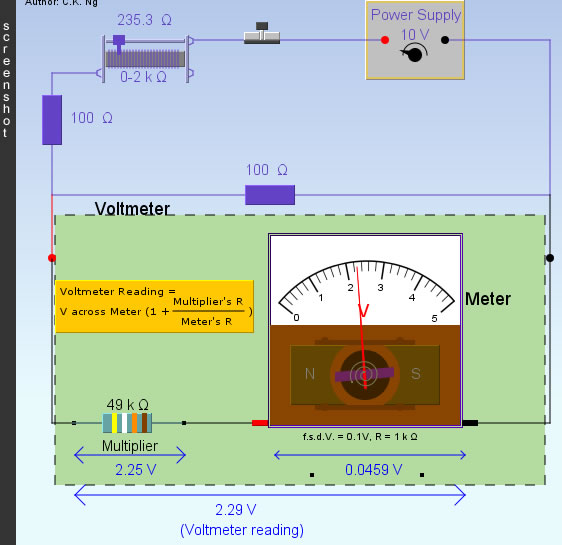Electric Meter (Shunts and Multipliers)

• "f.s.d.V. " stands for "full-scale-deflection voltage", it is the maximum voltage the meter can measure without the aid of an external resistor. The f.s.d.I. is f.s.d.V.divided by R.
• An ammeter can be converted from a meter of small full-scale-deflection current by connecting a small resistor in parallel with it. This small resistor is known as a shunt. A large portion of the current being measured will then flow through the shunt.
• A voltmeter can be converted from a meter of small full-scale-deflection voltage by connecting a large resistor in series with it. This large resistor is known as a multiplier. A large portion of the voltage being measured will then drop across the multiplier.

 Internal Link : Simple Electric Circuit

 The author (Chiu-king Ng) has the copyright on all the simulations in this website. Email phyAA@phy.hk, where AA is the prime number following 7. Last Update:2019-3-20